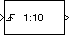Triggered Signal From Workspace

Import signal samples from MATLAB workspace when triggered

• Library:
• DSP System Toolbox / Signal Operations

•Description

The Triggered Signal From Workspace block imports signal samples from the MATLAB® workspace into the Simulink® model when triggered by the control signal at the input port (). The Signal parameter specifies the name of a MATLAB workspace variable containing the signal to import, or any valid MATLAB expression defining a matrix or 3-D array.

Ports

Input

expand all

Triggering input signal, specified as a scalar, vector, or matrix. This port is labelled with.

Data Types: single | double | int8 | int16 | int32 | int64 | uint8 | uint16 | uint32 | uint64 | Boolean | fixed point

Output

expand all

The block outputs the signal imported from the workspace when triggered by the control signal at the input port.

When the Signal parameter specifies an M-by-N matrix (M≠1), each of the N columns is treated as a distinct channel. You specify the frame size in the Samples per frame parameter, Mo. When triggered, the block outputs an Mo-by-N matrix containing Mo consecutive samples from each signal channel. For convenience, an imported row vector (M=1) is treated as a single channel, so the output dimension is Mo-by-1.

When the Signal parameter specifies an M-by-N-by-P array, the block generates a single page of the array (an M-by-N matrix) at each trigger time. The Samples per frame parameter must be set to 1.

Data Types: single | double | int8 | int16 | int32 | int64 | uint8 | uint16 | uint32 | uint64 | Boolean | fixed point

Parameters

expand all

The name of the MATLAB workspace variable from which to import the signal, or a valid MATLAB expression specifying the signal.

When the Signal parameter specifies an M-by-N matrix (M≠1), each of the N columns is treated as a distinct channel. You specify the frame size in the Samples per frame parameter, Mo. When triggered, the block outputs an Mo-by-N matrix containing Mo consecutive samples from each signal channel. For convenience, an imported row vector (M=1) is treated as a single channel, so the output dimension is Mo-by-1.

When the Signal parameter specifies an M-by-N-by-P array, the block generates a single page of the array (an M-by-N matrix) at each trigger time. The Samples per frame parameter must be set to 1.

The type of event that triggers the block to execute.

• Rising edge triggers execution of the block when the trigger input rises from a negative value to zero or a positive value, or from zero to a positive value.

• Falling edge triggers execution of the block when the trigger input falls from a positive value to zero or a negative value, or from zero to a negative value.

• Either edge triggers execution of the block when either a rising or falling edge occurs.

The value to output until the first trigger event is received. Between trigger events, the block holds the output value constant at its most recent value (that is, no linear interpolation takes place). For single-channel signals, the Initial output can be a vector of length Mo or a scalar to repeat across the Mo elements of the initial output frames. For matrix outputs (Mo-by-N or M-by-N), the Initial output parameter value can be a matrix of the same size or a scalar to be repeated across all elements of the initial output.

The number of samples, Mo, to buffer into each output frame specified as a positive integer scalar. This value must be 1 when you specify a 3-D array in the Signal parameter.

Specifies the output after all of the specified signal samples have been generated.

• When you specify Setting To Zero, the block generates zero-valued outputs for the duration of the simulation after generating the last frame of the signal.

• When you specify Holding Final Value, the block repeats the final sample for the duration of the simulation after generating the last frame of the signal.

• When you specify Cyclic Repetition, the block repeats the signal from the beginning after generating the last frame. When there are not enough samples at the end of the signal to fill the final frame, the block zero-pads the final frame as necessary to ensure that the output for each cycle is identical. For example, the ith frame of one cycle contains the same samples as the ith frame of any other cycle.

The block does not extrapolate the imported signal beyond the last sample.

Block Characteristics

 Data Types double | fixed point | integer | single Direct Feedthrough no Multidimensional Signals no Variable-Size Signals no Zero-Crossing Detection no

Extended Capabilities

Fixed-Point ConversionDesign and simulate fixed-point systems using Fixed-Point Designer™.